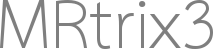# Representing diffusion parameters as math formulas

Hi everyone!

This is a bit off-topic, but I thought that some of you could confirm my thoughts on this. I am writing a paper and I use the formulas for the tensor-derived metrics fractional anisotropy, mean and radial diffusivity. I am requested to insert them as math formulas and not as images. Most papers do not allow copying/pasting from the formulas they have used so instead, I used the ‘edit’ option from the Wikipedia articles for these quantities and took the formulas from there.

\mathrm{MD} = (\lambda_1 + \lambda_2 + \lambda_3) /3 \qquad \text{(MD formula)} \\ \text{FA} = \sqrt{\frac{1}{2}} \frac{\sqrt{(\lambda_1 - \lambda_2)^2 + (\lambda_2 - \lambda_3)^2 + (\lambda_3 - \lambda_1)^2}}{\sqrt{\lambda_1^2 + \lambda_2^2 + \lambda_3^2}} \qquad \text{(FA formula)} \\ \lambda_{\perp} = (\lambda_2 + \lambda_3)/2 . \qquad \text{(RD formula)}

Has someone done that before or has found another website/way to derive formulas for tensor quantities?

Thank you very much for your opinions!Ivana

Hi, is your question about how to insert these equations into your manuscript? Or whether these equations are correct? If the latter, they seem OK to me. If the former, that depends on the software you’re using to write your manuscript. Word has a decent equation editor, or if you use LaTeX, your equations are actually already in the right format for that. I’ve edited your post to show you what they look like (you can actually typeset LaTeX equations on this forum by enclosing them in ). If the issue is that you’re trying to copy/paste these formulae into Word, then I don’t know a way of doing this, but these are short enough that you can trivially type them into the equation editor.

If I’ve got the wrong end of the stick, feel free to let me know!

Hello MRTrix support team,

Thank you for your reply. Indeed, that’s the confirmation I was looking forKind regards,
Ivana